Скачать презентацию Examples on using Psychart — The digital psychrometric

9878302af4dcdf460b6444dce9beafd0.ppt

• Количество слайдов: 49Examples on using Psychart -- The digital psychrometric software Wei Fang, Ph. D. , Professor Dept. of Bio-Industrial Mechatronics Engineering National Taiwan University Email: [email protected] edu. tw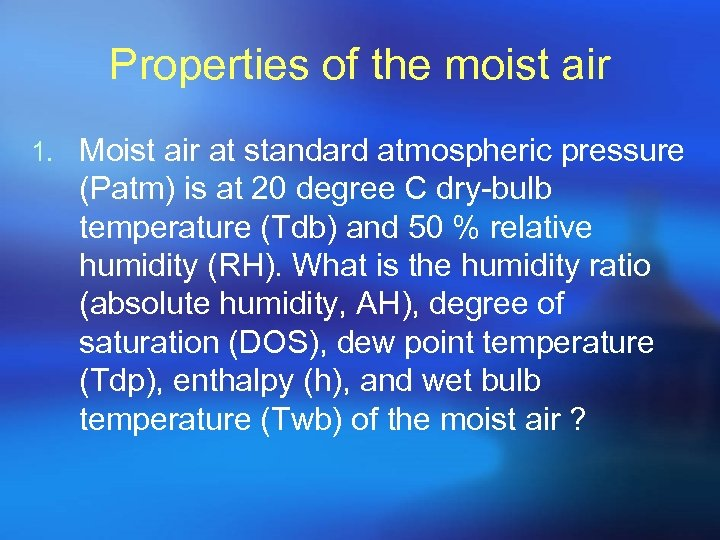Properties of the moist air 1. Moist air at standard atmospheric pressure (Patm) is at 20 degree C dry-bulb temperature (Tdb) and 50 % relative humidity (RH). What is the humidity ratio (absolute humidity, AH), degree of saturation (DOS), dew point temperature (Tdp), enthalpy (h), and wet bulb temperature (Twb) of the moist air ?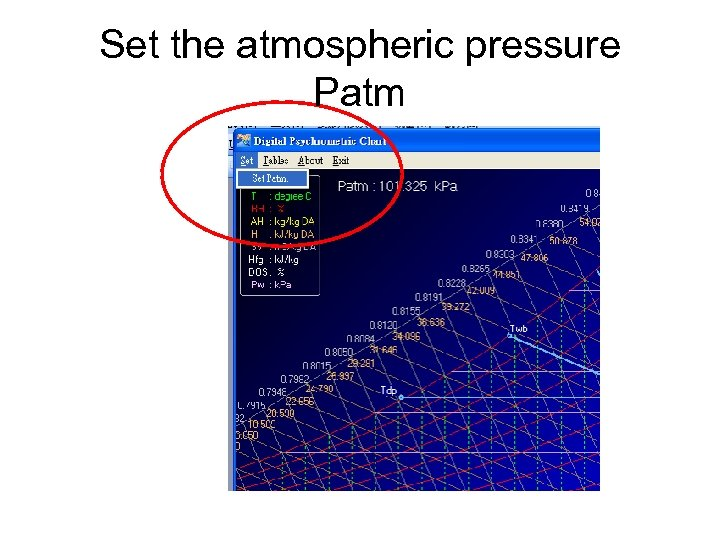Set the atmospheric pressure Patm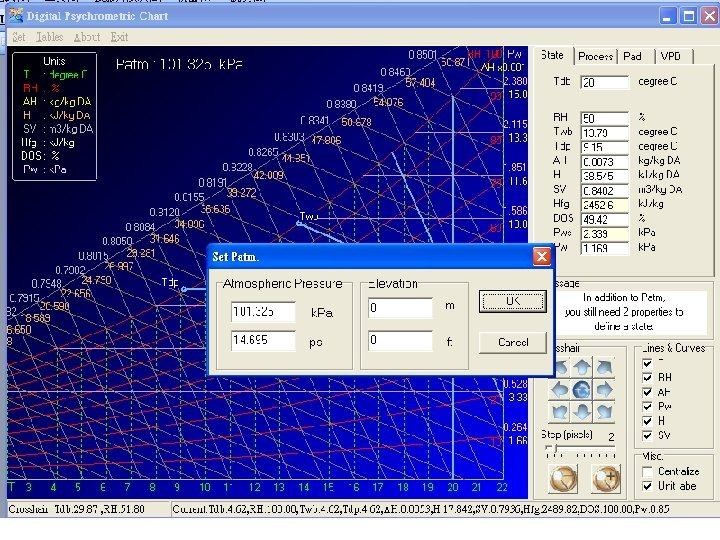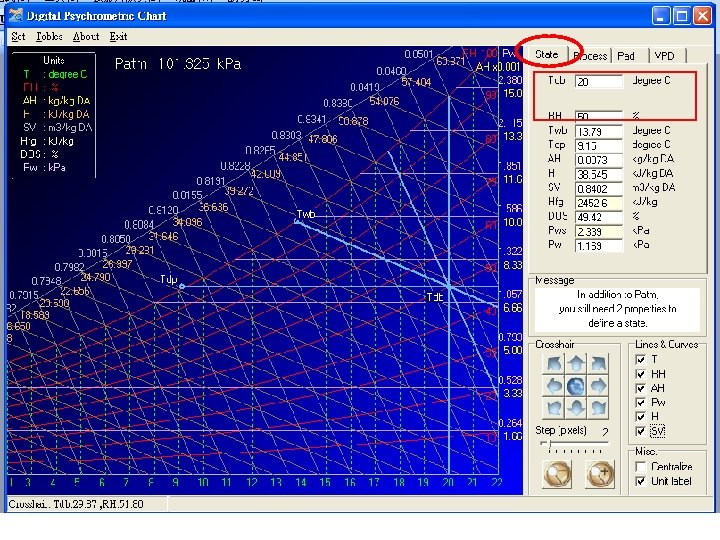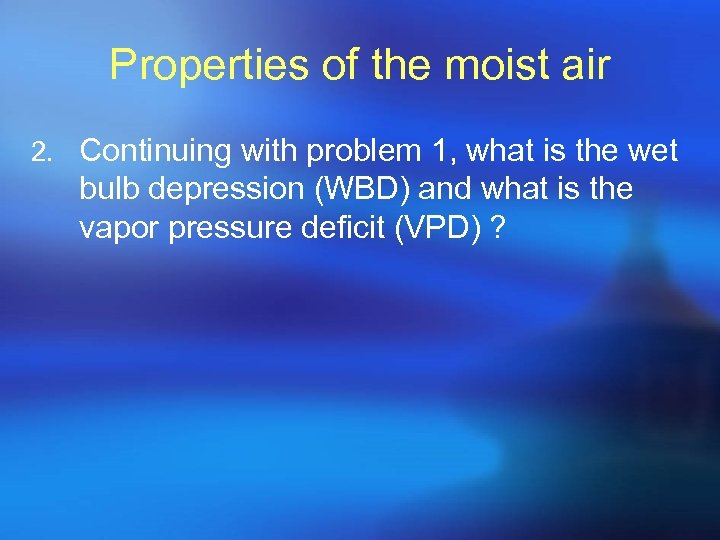Properties of the moist air 2. Continuing with problem 1, what is the wet bulb depression (WBD) and what is the vapor pressure deficit (VPD) ?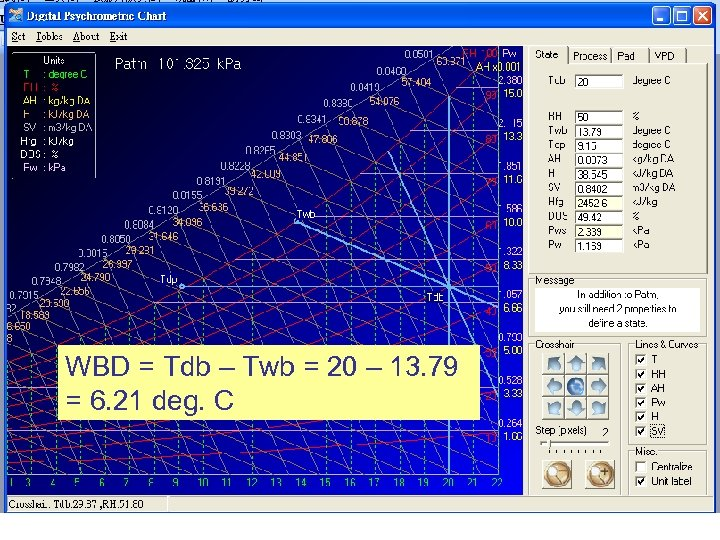WBD = Tdb – Twb = 20 – 13. 79 = 6. 21 deg. C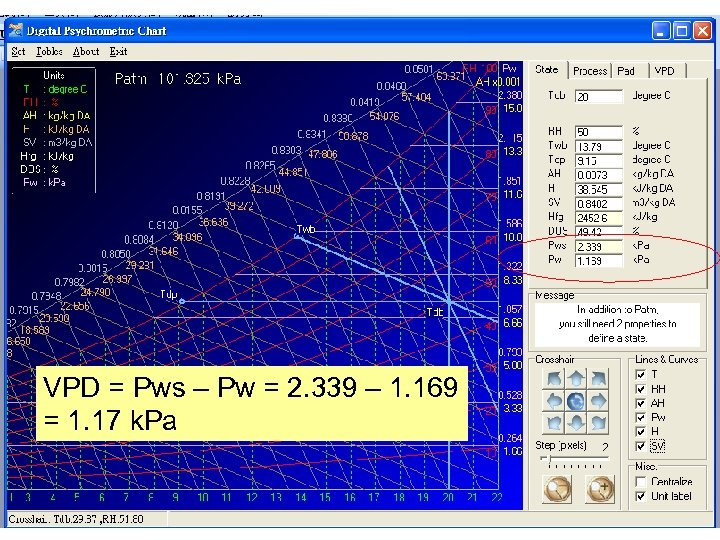VPD = Pws – Pw = 2. 339 – 1. 169 = 1. 17 k. Pa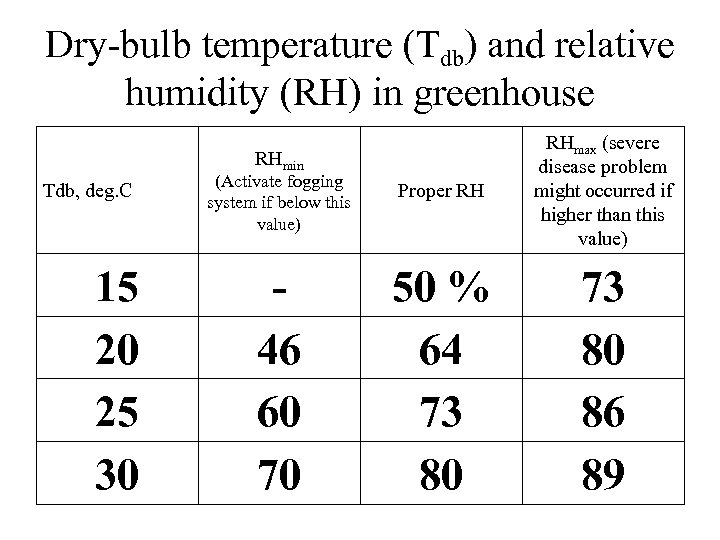Dry-bulb temperature (Tdb) and relative humidity (RH) in greenhouse (Activate fogging system if below this value) Proper RH RHmax (severe disease problem might occurred if higher than this value) 46 60 70 50 % 64 73 80 86 89 RHmin Tdb, deg. C 15 20 25 30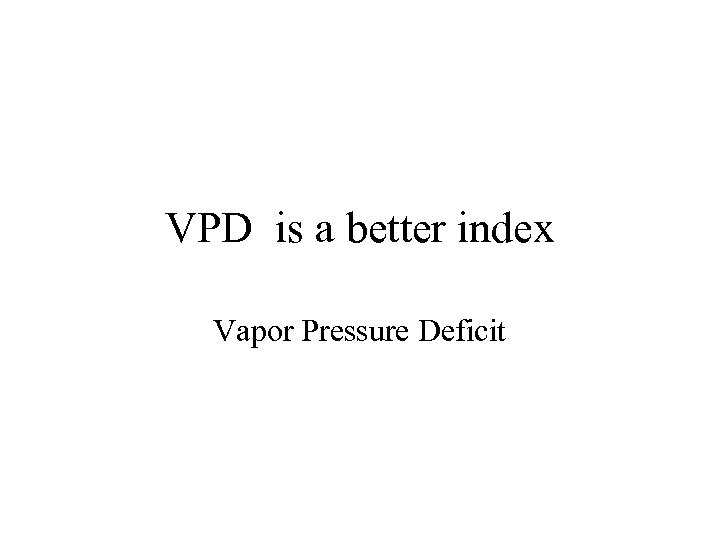VPD is a better index Vapor Pressure Deficit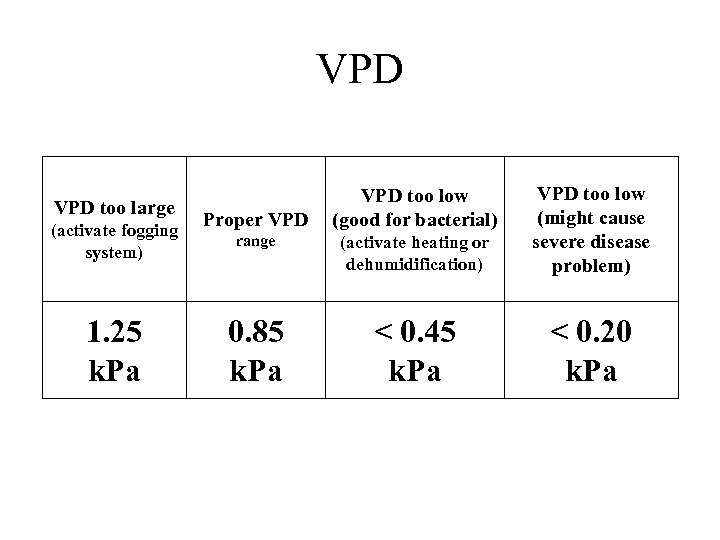VPD too large (activate fogging system) 1. 25 k. Pa range (activate heating or dehumidification) VPD too low (might cause severe disease problem) 0. 85 k. Pa < 0. 45 k. Pa < 0. 20 k. Pa Proper VPD too low (good for bacterial)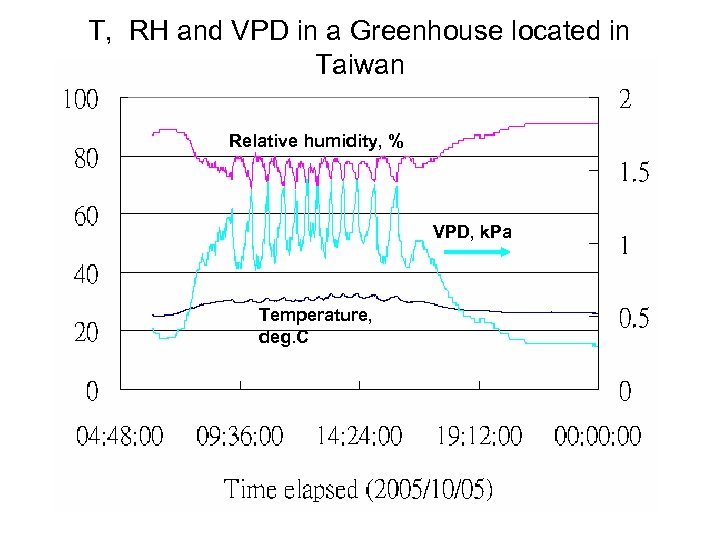T, RH and VPD in a Greenhouse located in Taiwan Relative humidity, % VPD, k. Pa Temperature, deg. C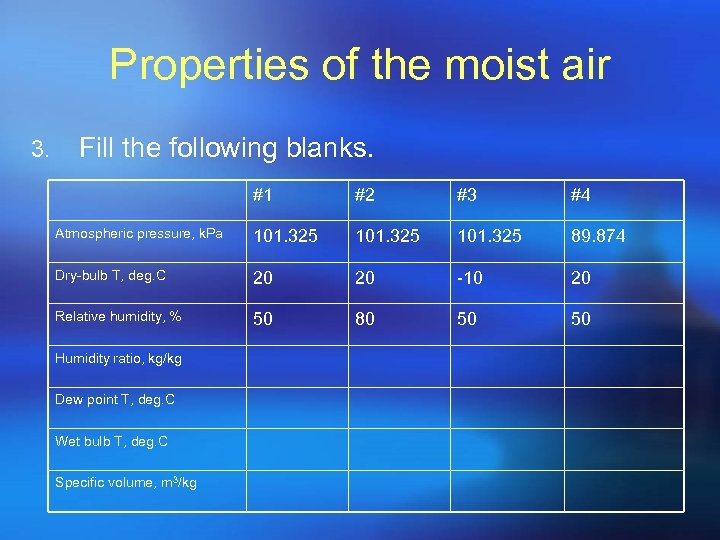Properties of the moist air 3. Fill the following blanks. #1 #2 #3 #4 Atmospheric pressure, k. Pa 101. 325 89. 874 Dry-bulb T, deg. C 20 20 -10 20 Relative humidity, % 50 80 50 50 Humidity ratio, kg/kg Dew point T, deg. C Wet bulb T, deg. C Specific volume, m 3/kg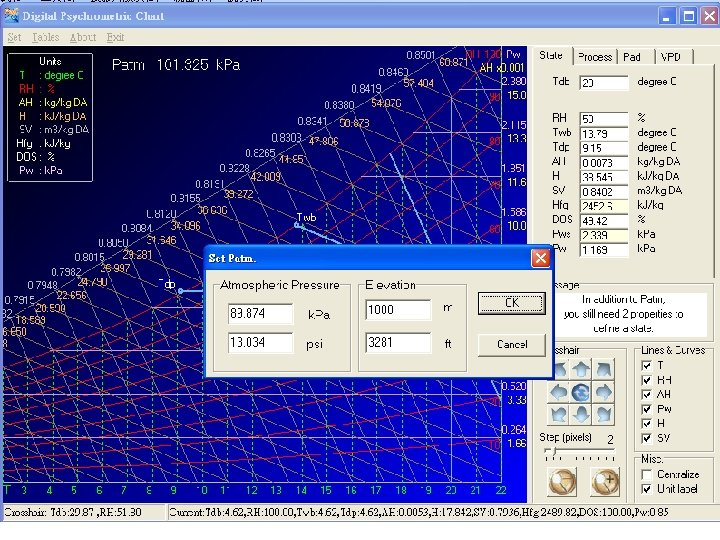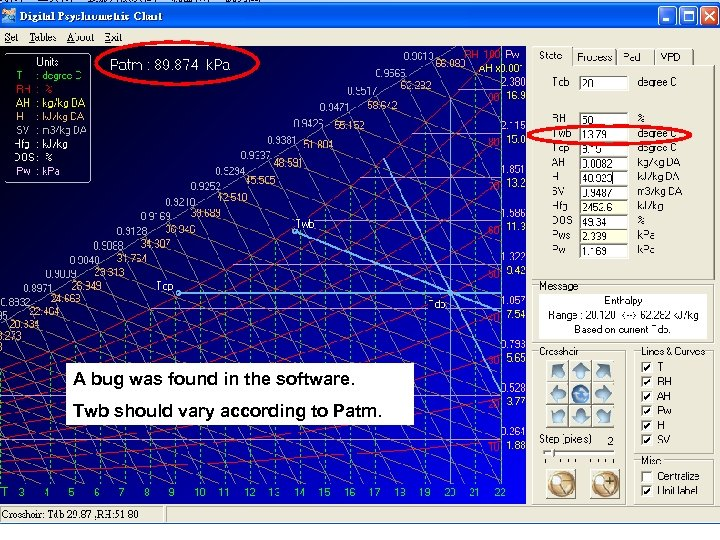A bug was found in the software. Twb should vary according to Patm.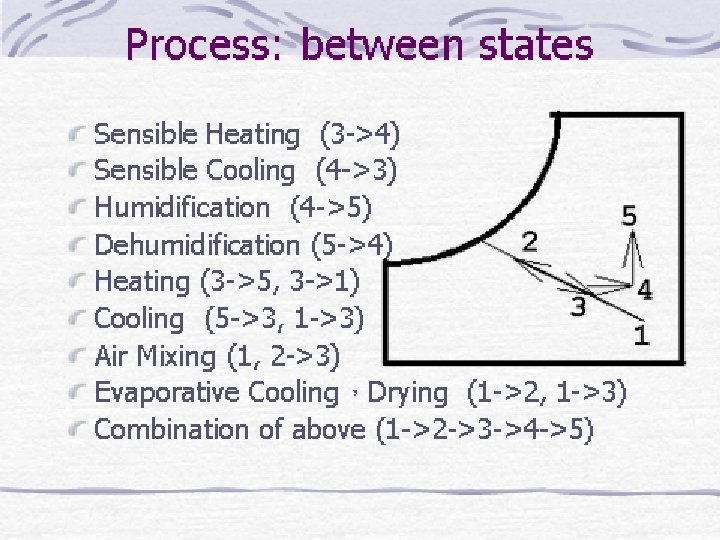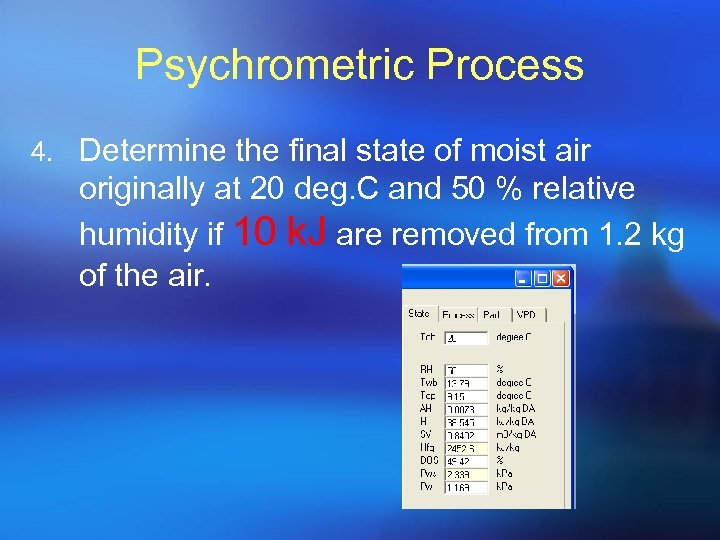Psychrometric Process 4. Determine the final state of moist air originally at 20 deg. C and 50 % relative humidity if 10 k. J are removed from 1. 2 kg of the air.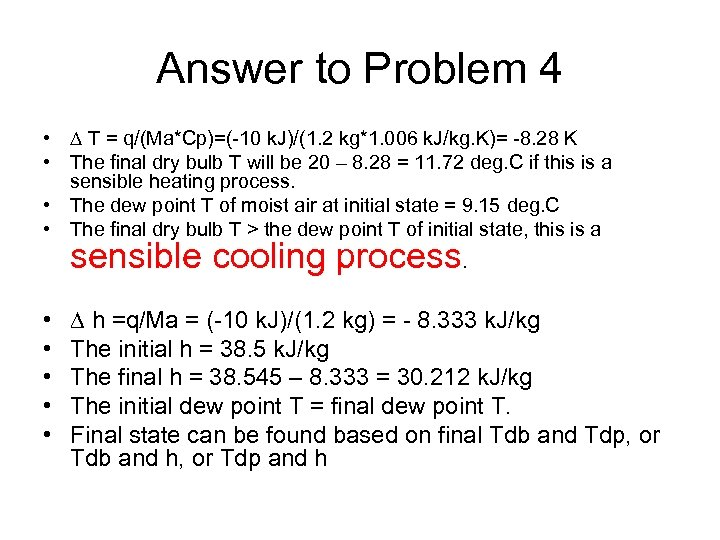Answer to Problem 4 • T = q/(Ma*Cp)=(-10 k. J)/(1. 2 kg*1. 006 k. J/kg. K)= -8. 28 K • The final dry bulb T will be 20 – 8. 28 = 11. 72 deg. C if this is a sensible heating process. • The dew point T of moist air at initial state = 9. 15 deg. C • The final dry bulb T > the dew point T of initial state, this is a sensible cooling process. • • • h =q/Ma = (-10 k. J)/(1. 2 kg) = - 8. 333 k. J/kg The initial h = 38. 5 k. J/kg The final h = 38. 545 – 8. 333 = 30. 212 k. J/kg The initial dew point T = final dew point T. Final state can be found based on final Tdb and Tdp, or Tdb and h, or Tdp and h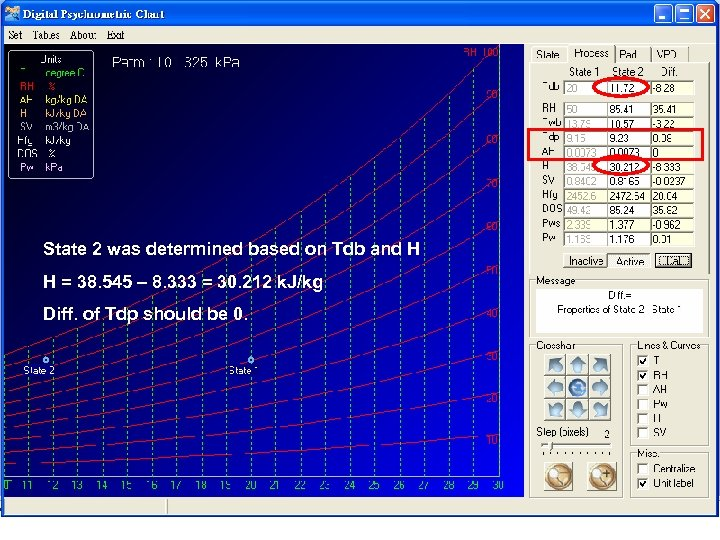State 2 was determined based on Tdb and H H = 38. 545 – 8. 333 = 30. 212 k. J/kg Diff. of Tdp should be 0.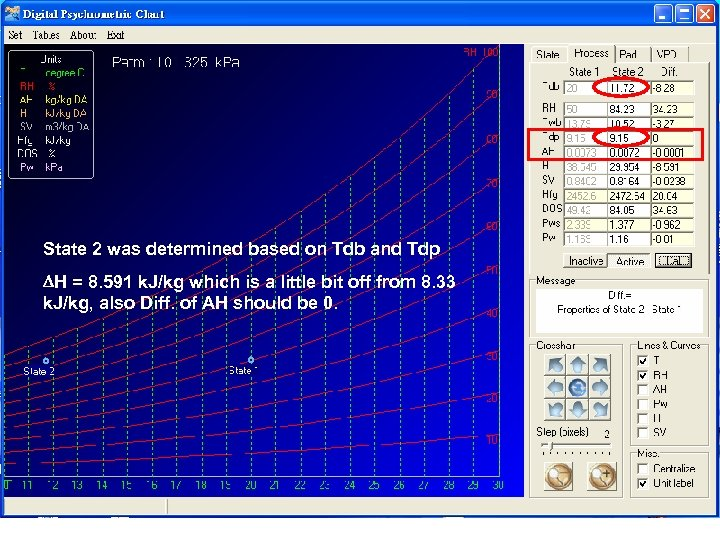State 2 was determined based on Tdb and Tdp H = 8. 591 k. J/kg which is a little bit off from 8. 33 k. J/kg, also Diff. of AH should be 0.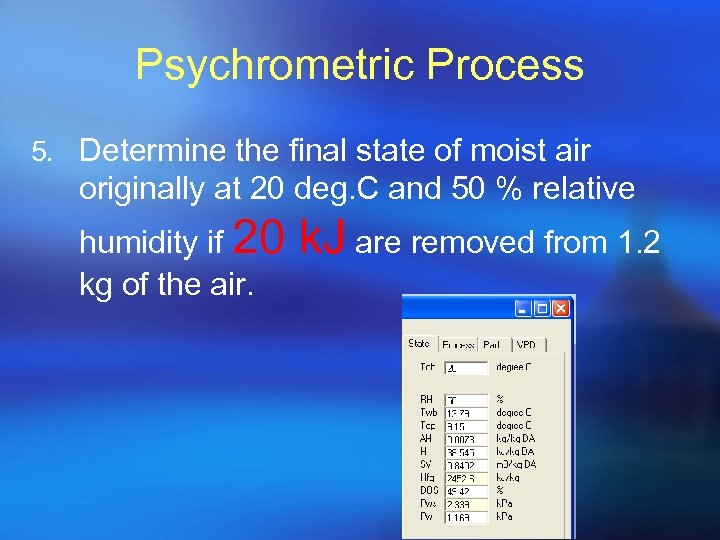Psychrometric Process 5. Determine the final state of moist air originally at 20 deg. C and 50 % relative humidity if 20 kg of the air. k. J are removed from 1. 2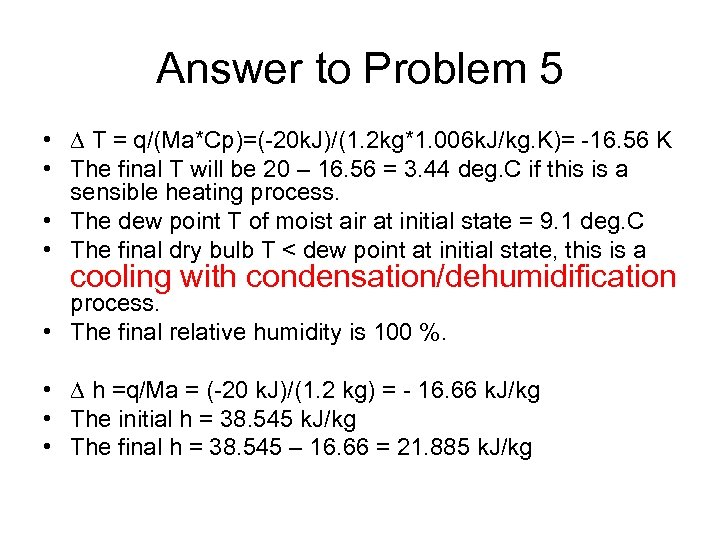Answer to Problem 5 • T = q/(Ma*Cp)=(-20 k. J)/(1. 2 kg*1. 006 k. J/kg. K)= -16. 56 K • The final T will be 20 – 16. 56 = 3. 44 deg. C if this is a sensible heating process. • The dew point T of moist air at initial state = 9. 1 deg. C • The final dry bulb T < dew point at initial state, this is a cooling with condensation/dehumidification process. • The final relative humidity is 100 %. • h =q/Ma = (-20 k. J)/(1. 2 kg) = - 16. 66 k. J/kg • The initial h = 38. 545 k. J/kg • The final h = 38. 545 – 16. 66 = 21. 885 k. J/kg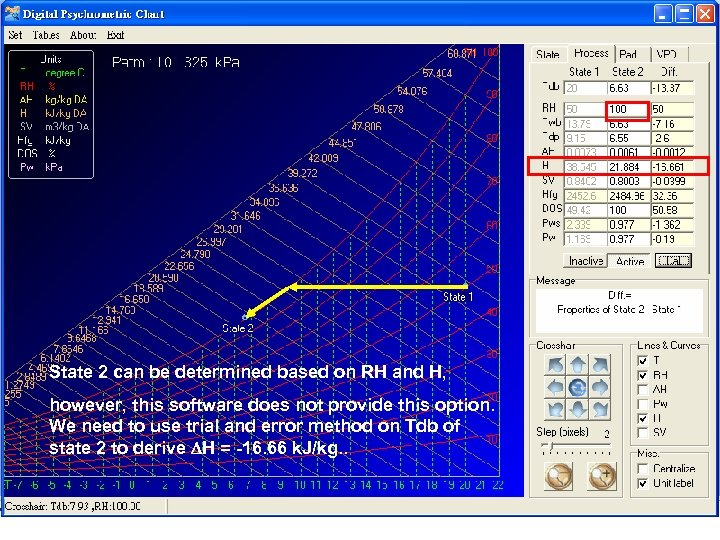State 2 can be determined based on RH and H, however, this software does not provide this option. We need to use trial and error method on Tdb of state 2 to derive H = -16. 66 k. J/kg. .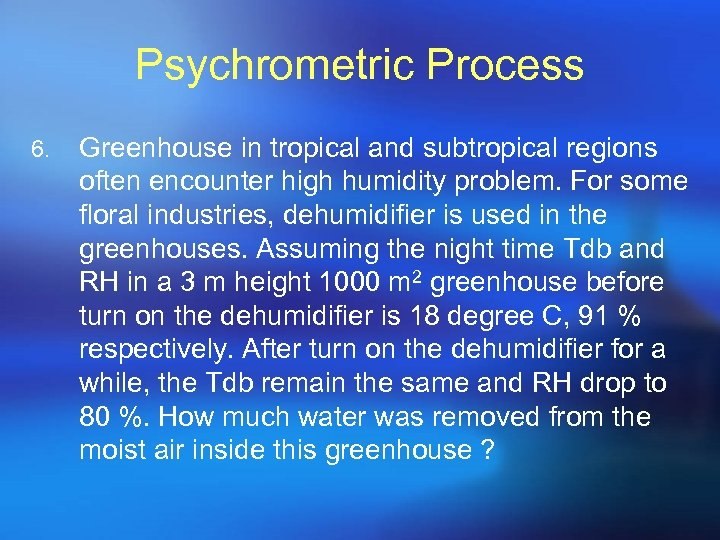Psychrometric Process 6. Greenhouse in tropical and subtropical regions often encounter high humidity problem. For some floral industries, dehumidifier is used in the greenhouses. Assuming the night time Tdb and RH in a 3 m height 1000 m 2 greenhouse before turn on the dehumidifier is 18 degree C, 91 % respectively. After turn on the dehumidifier for a while, the Tdb remain the same and RH drop to 80 %. How much water was removed from the moist air inside this greenhouse ?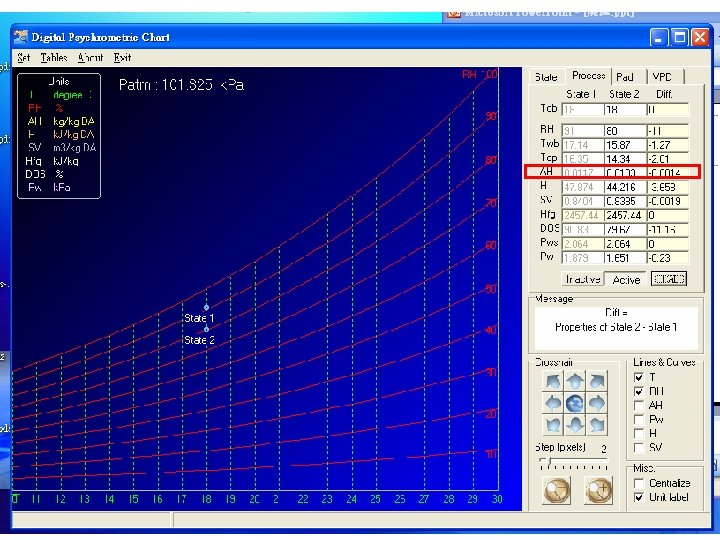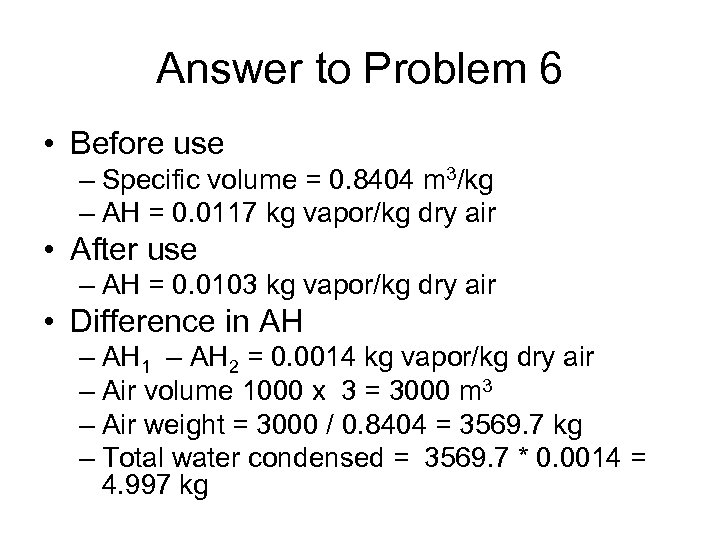Answer to Problem 6 • Before use – Specific volume = 0. 8404 m 3/kg – AH = 0. 0117 kg vapor/kg dry air • After use – AH = 0. 0103 kg vapor/kg dry air • Difference in AH – AH 1 – AH 2 = 0. 0014 kg vapor/kg dry air – Air volume 1000 x 3 = 3000 m 3 – Air weight = 3000 / 0. 8404 = 3569. 7 kg – Total water condensed = 3569. 7 * 0. 0014 = 4. 997 kg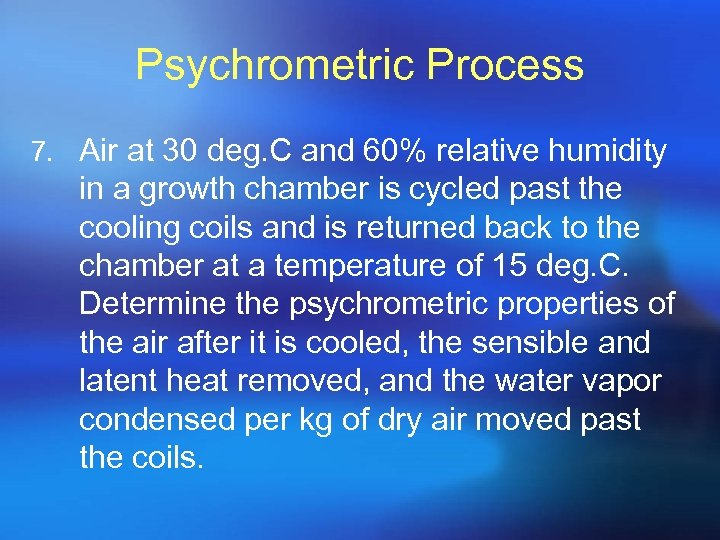Psychrometric Process 7. Air at 30 deg. C and 60% relative humidity in a growth chamber is cycled past the cooling coils and is returned back to the chamber at a temperature of 15 deg. C. Determine the psychrometric properties of the air after it is cooled, the sensible and latent heat removed, and the water vapor condensed per kg of dry air moved past the coils.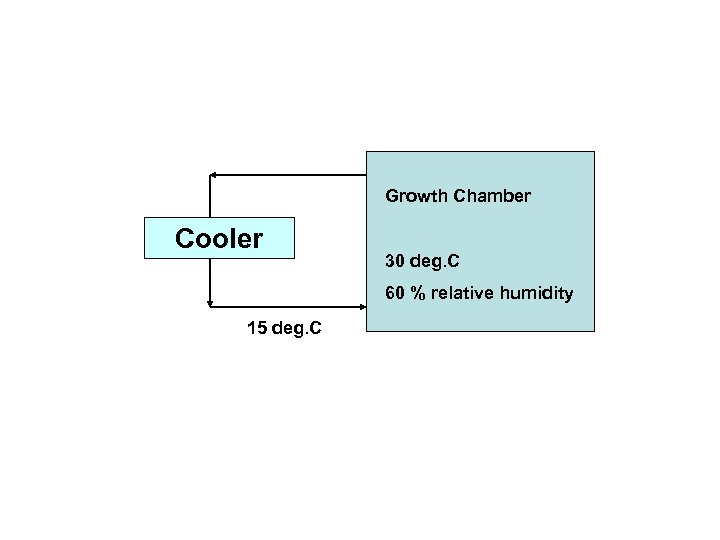Growth Chamber Cooler 30 deg. C 60 % relative humidity 15 deg. C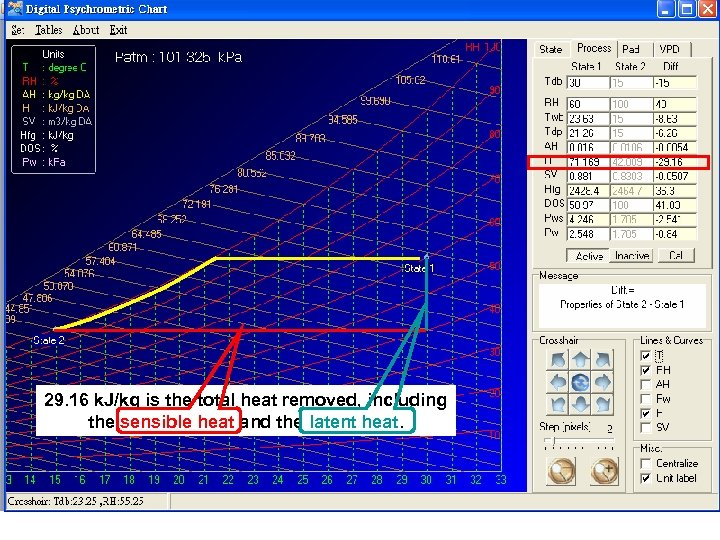29. 16 k. J/kg is the total heat removed, including the sensible heat and the latent heat.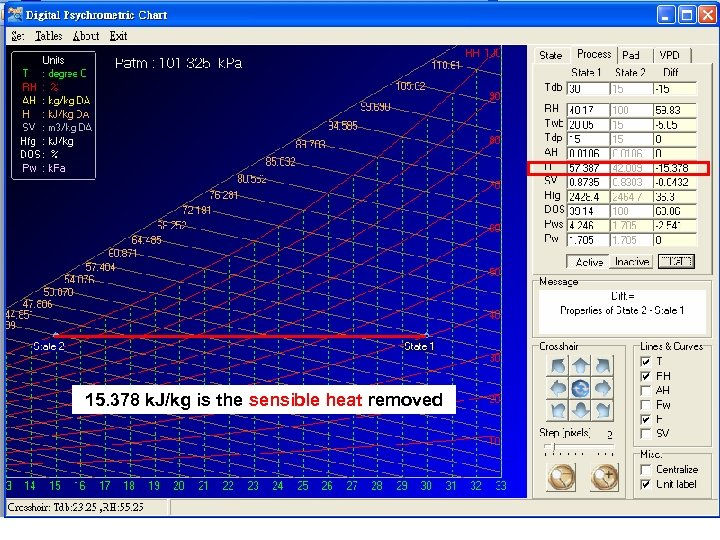15. 378 k. J/kg is the sensible heat removed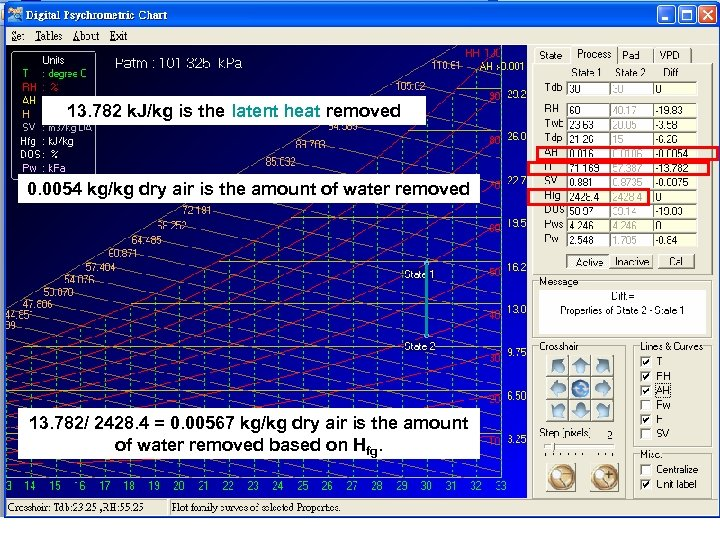13. 782 k. J/kg is the latent heat removed 0. 0054 kg/kg dry air is the amount of water removed 13. 782/ 2428. 4 = 0. 00567 kg/kg dry air is the amount of water removed based on Hfg.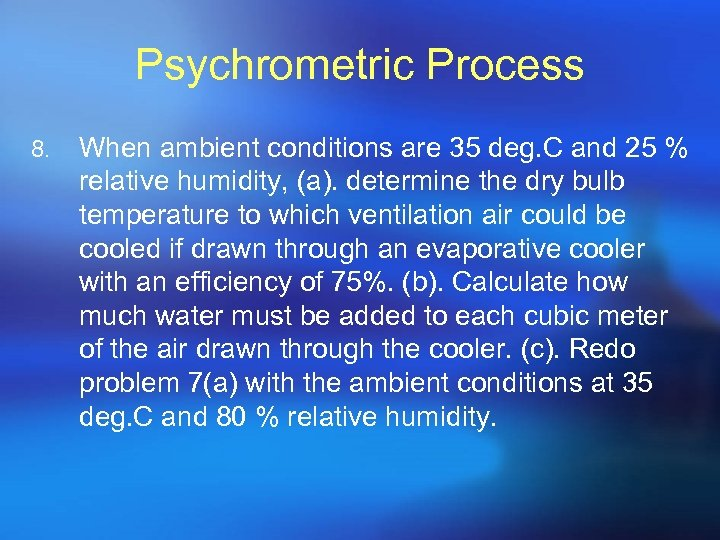Psychrometric Process 8. When ambient conditions are 35 deg. C and 25 % relative humidity, (a). determine the dry bulb temperature to which ventilation air could be cooled if drawn through an evaporative cooler with an efficiency of 75%. (b). Calculate how much water must be added to each cubic meter of the air drawn through the cooler. (c). Redo problem 7(a) with the ambient conditions at 35 deg. C and 80 % relative humidity.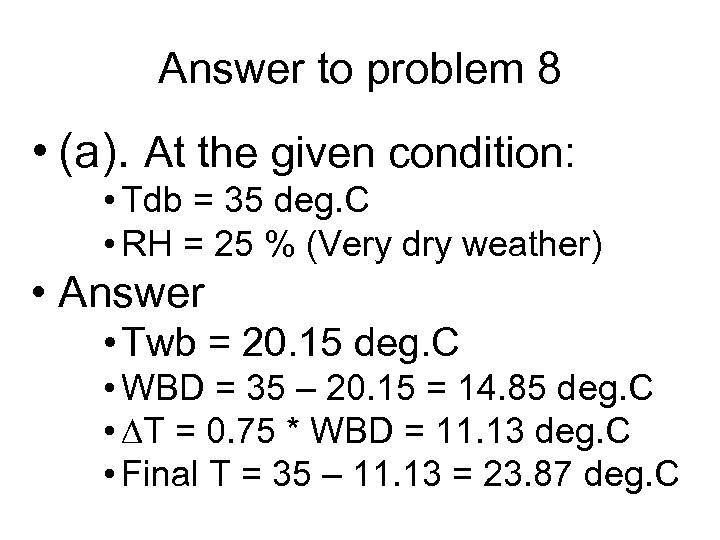Answer to problem 8 • (a). At the given condition: • Tdb = 35 deg. C • RH = 25 % (Very dry weather) • Answer • Twb = 20. 15 deg. C • WBD = 35 – 20. 15 = 14. 85 deg. C • T = 0. 75 * WBD = 11. 13 deg. C • Final T = 35 – 11. 13 = 23. 87 deg. C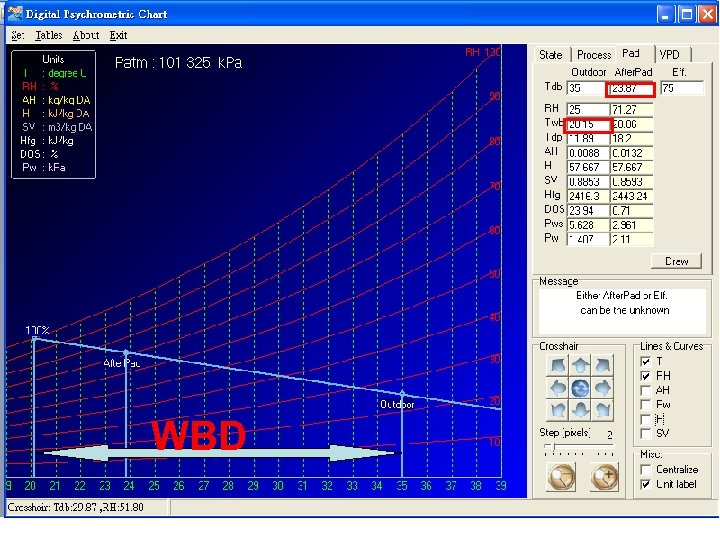WBD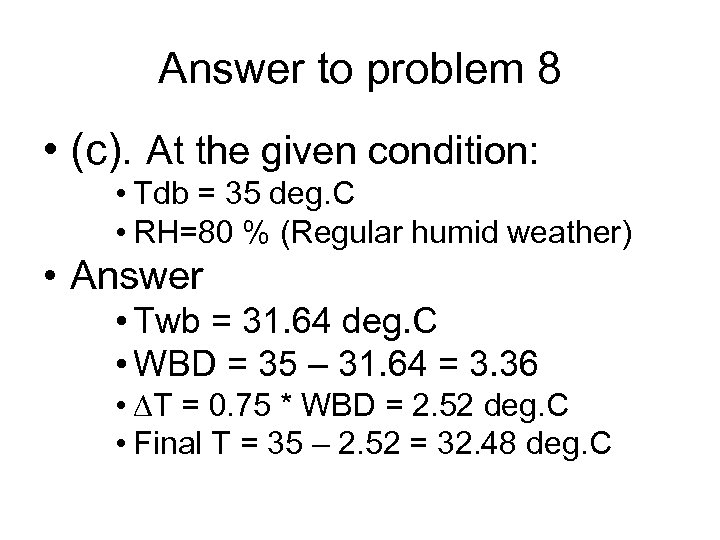Answer to problem 8 • (c). At the given condition: • Tdb = 35 deg. C • RH=80 % (Regular humid weather) • Answer • Twb = 31. 64 deg. C • WBD = 35 – 31. 64 = 3. 36 • T = 0. 75 * WBD = 2. 52 deg. C • Final T = 35 – 2. 52 = 32. 48 deg. C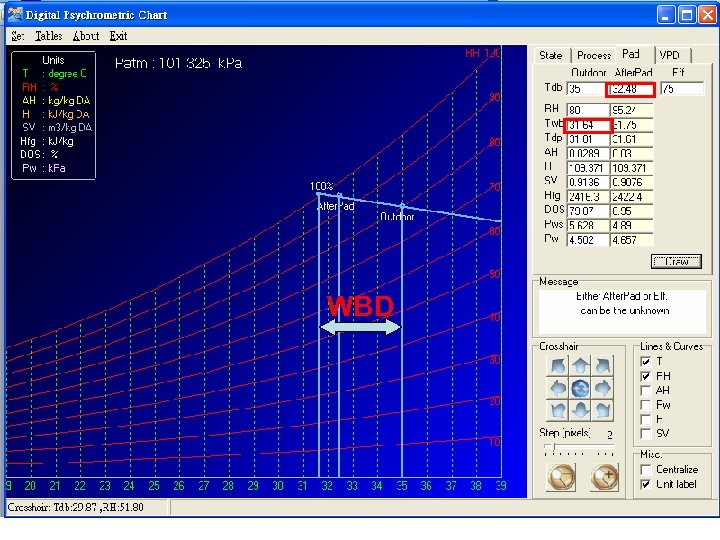WBD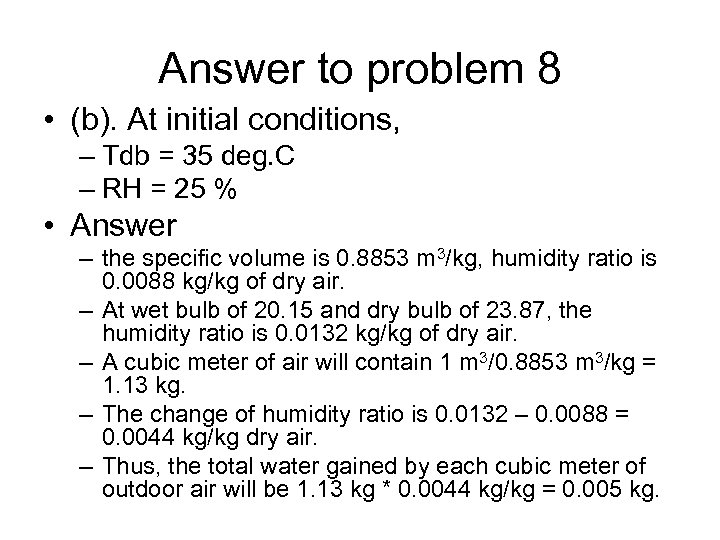Answer to problem 8 • (b). At initial conditions, – Tdb = 35 deg. C – RH = 25 % • Answer – the specific volume is 0. 8853 m 3/kg, humidity ratio is 0. 0088 kg/kg of dry air. – At wet bulb of 20. 15 and dry bulb of 23. 87, the humidity ratio is 0. 0132 kg/kg of dry air. – A cubic meter of air will contain 1 m 3/0. 8853 m 3/kg = 1. 13 kg. – The change of humidity ratio is 0. 0132 – 0. 0088 = 0. 0044 kg/kg dry air. – Thus, the total water gained by each cubic meter of outdoor air will be 1. 13 kg * 0. 0044 kg/kg = 0. 005 kg.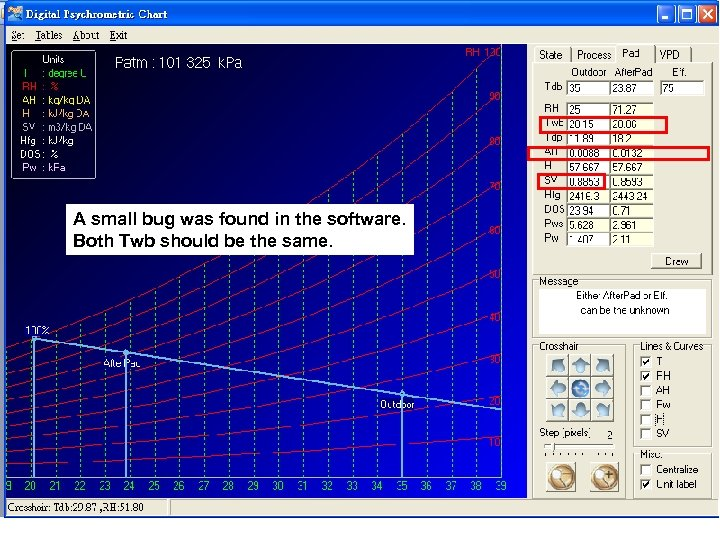A small bug was found in the software. Both Twb should be the same.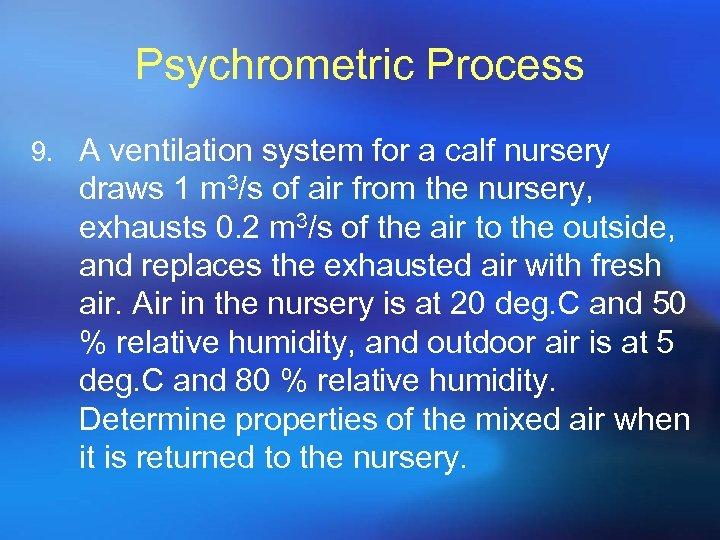Psychrometric Process 9. A ventilation system for a calf nursery draws 1 m 3/s of air from the nursery, exhausts 0. 2 m 3/s of the air to the outside, and replaces the exhausted air with fresh air. Air in the nursery is at 20 deg. C and 50 % relative humidity, and outdoor air is at 5 deg. C and 80 % relative humidity. Determine properties of the mixed air when it is returned to the nursery.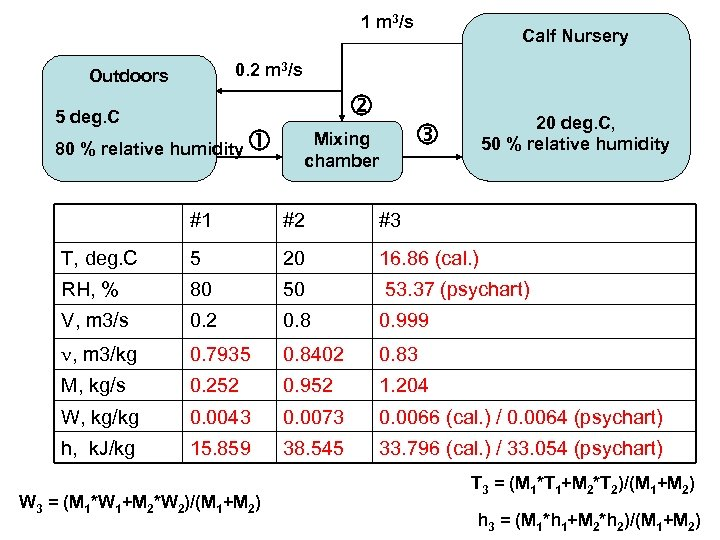1 m 3/s Calf Nursery 0. 2 m 3/s Outdoors 5 deg. C 80 % relative humidity 20 deg. C, 50 % relative humidity Mixing chamber #1 #2 #3 T, deg. C 5 20 16. 86 (cal. ) RH, % 80 50 53. 37 (psychart) V, m 3/s 0. 2 0. 8 0. 999 , m 3/kg 0. 7935 0. 8402 0. 83 M, kg/s 0. 252 0. 952 1. 204 W, kg/kg 0. 0043 0. 0073 0. 0066 (cal. ) / 0. 0064 (psychart) h, k. J/kg 15. 859 38. 545 33. 796 (cal. ) / 33. 054 (psychart) W 3 = (M 1*W 1+M 2*W 2)/(M 1+M 2) T 3 = (M 1*T 1+M 2*T 2)/(M 1+M 2) h 3 = (M 1*h 1+M 2*h 2)/(M 1+M 2)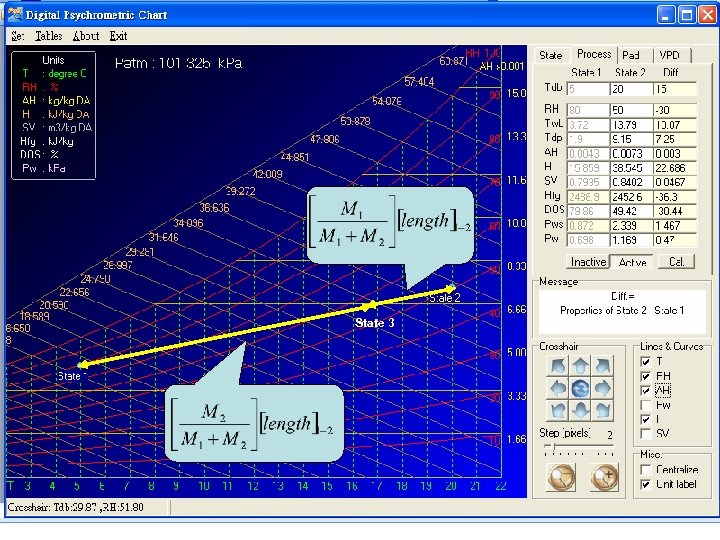State 3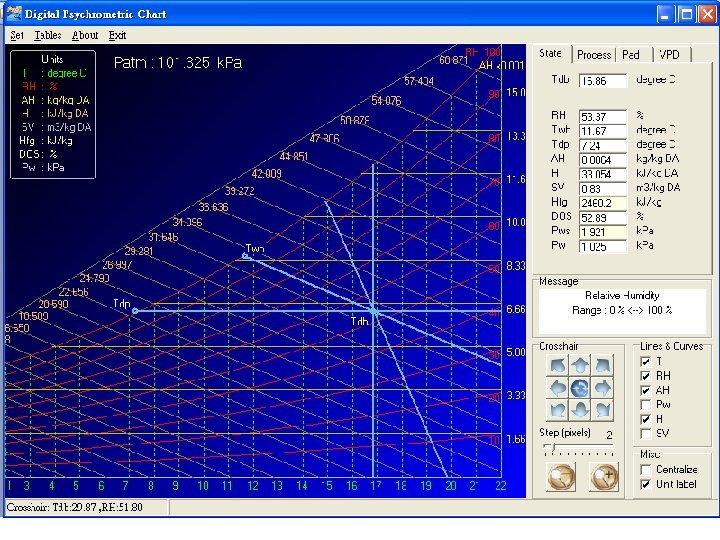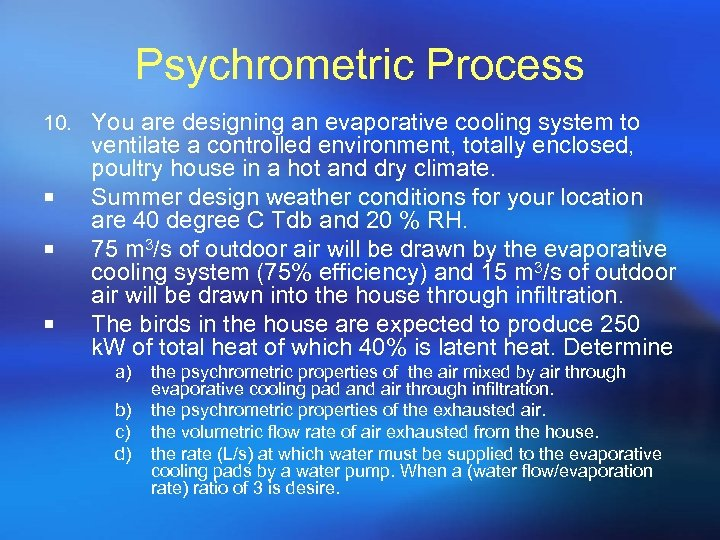Psychrometric Process 10. You are designing an evaporative cooling system to ¡ ¡ ¡ ventilate a controlled environment, totally enclosed, poultry house in a hot and dry climate. Summer design weather conditions for your location are 40 degree C Tdb and 20 % RH. 75 m 3/s of outdoor air will be drawn by the evaporative cooling system (75% efficiency) and 15 m 3/s of outdoor air will be drawn into the house through infiltration. The birds in the house are expected to produce 250 k. W of total heat of which 40% is latent heat. Determine a) b) c) d) the psychrometric properties of the air mixed by air through evaporative cooling pad and air through infiltration. the psychrometric properties of the exhausted air. the volumetric flow rate of air exhausted from the house. the rate (L/s) at which water must be supplied to the evaporative cooling pads by a water pump. When a (water flow/evaporation rate) ratio of 3 is desire.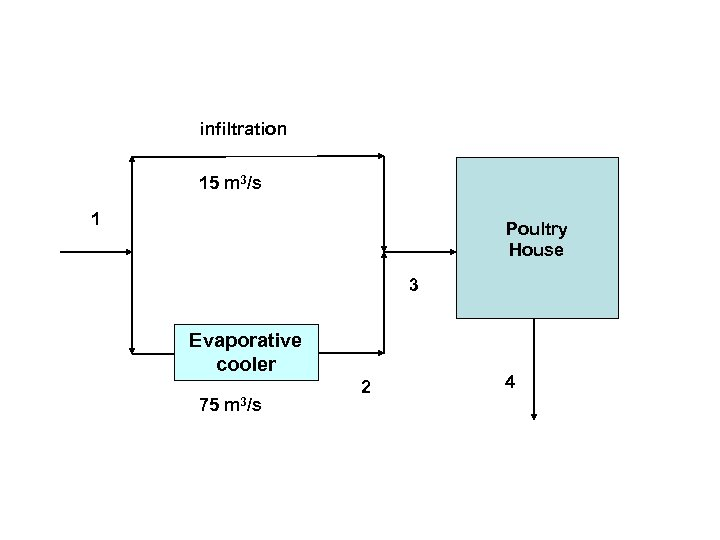infiltration 15 m 3/s 1 Poultry House 3 Evaporative cooler 75 m 3/s 2 4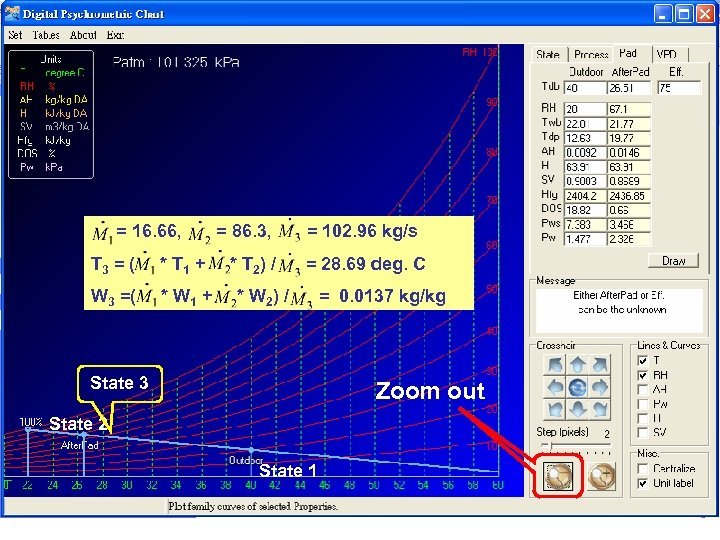= 16. 66, T 3 = ( * T 1 + W 3 =( * W 1 + = 86. 3, * T 2) / = 102. 96 kg/s = 28. 69 deg. C * W 2) / State 3 = 0. 0137 kg/kg Zoom out State 2 State 1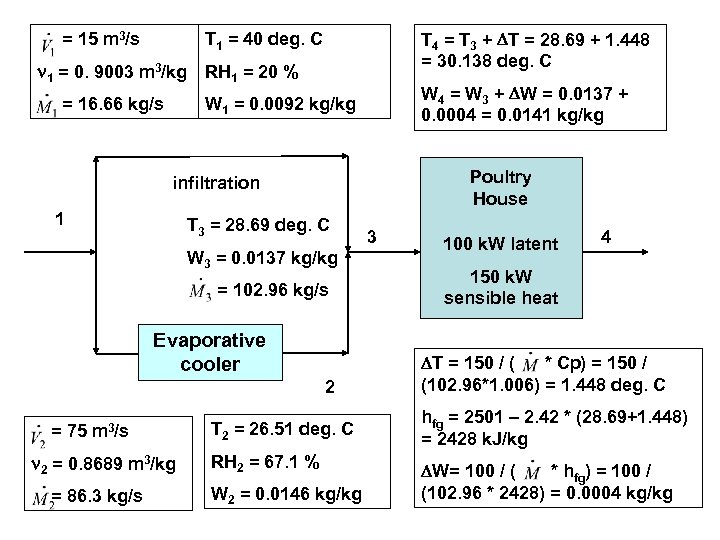= 15 m 3/s T 4 = T 3 + T = 28. 69 + 1. 448 = 30. 138 deg. C T 1 = 40 deg. C 1 = 0. 9003 m 3/kg RH 1 = 20 % = 16. 66 kg/s W 4 = W 3 + W = 0. 0137 + 0. 0004 = 0. 0141 kg/kg W 1 = 0. 0092 kg/kg Poultry House infiltration 1 T 3 = 28. 69 deg. C W 3 = 0. 0137 kg/kg = 102. 96 kg/s Evaporative cooler 2 = 75 m 3/s 2 = 0. 8689 m 3/kg = 86. 3 kg/s T 2 = 26. 51 deg. C RH 2 = 67. 1 % W 2 = 0. 0146 kg/kg 3 100 k. W latent 4 150 k. W sensible heat T = 150 / ( * Cp) = 150 / (102. 96*1. 006) = 1. 448 deg. C hfg = 2501 – 2. 42 * (28. 69+1. 448) = 2428 k. J/kg W= 100 / ( * hfg) = 100 / (102. 96 * 2428) = 0. 0004 kg/kg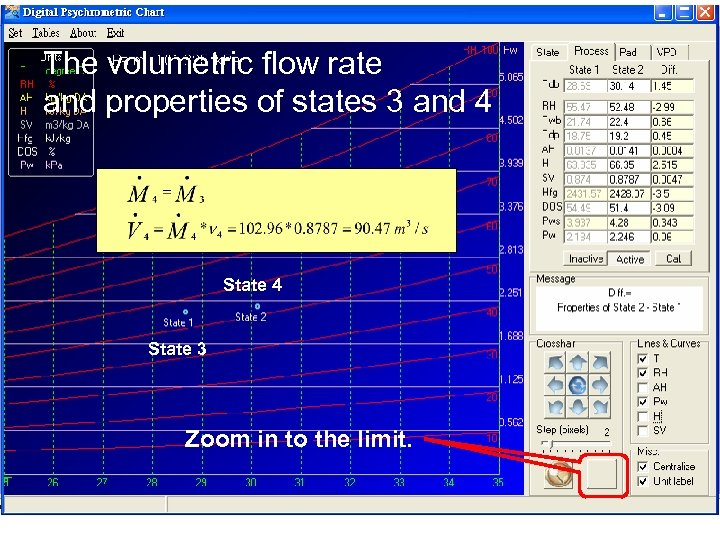The volumetric flow rate and properties of states 3 and 4 State 3 Zoom in to the limit.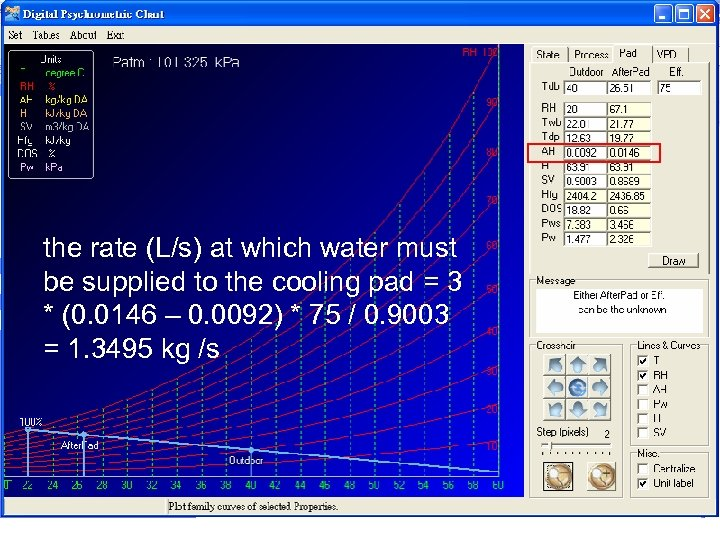the rate (L/s) at which water must be supplied to the cooling pad = 3 * (0. 0146 – 0. 0092) * 75 / 0. 9003 = 1. 3495 kg /s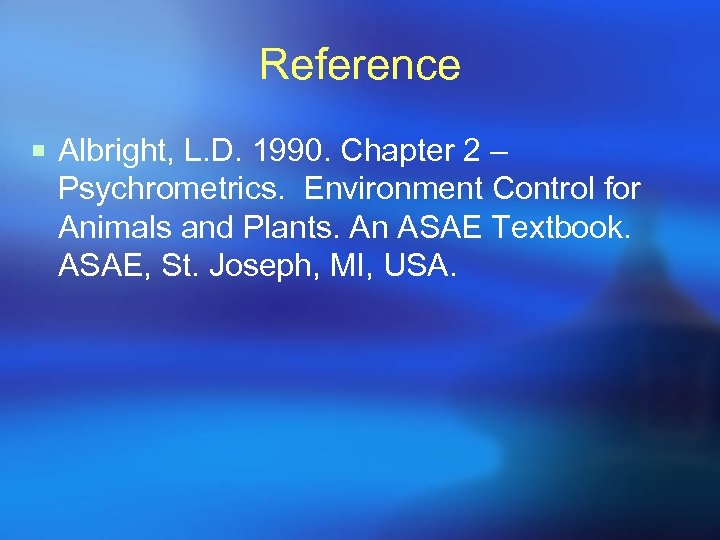Reference ¡ Albright, L. D. 1990. Chapter 2 – Psychrometrics. Environment Control for Animals and Plants. An ASAE Textbook. ASAE, St. Joseph, MI, USA.